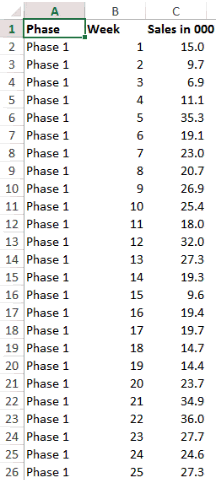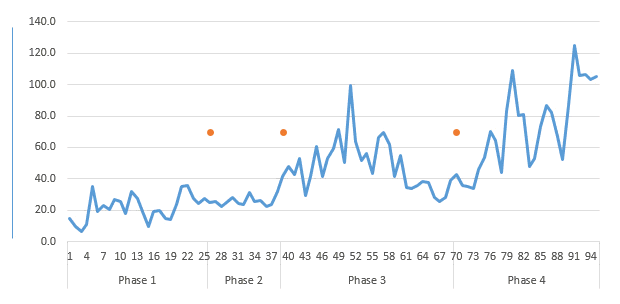Line charts are most often drawn for time series analysis and it would be interesting to divide our line chart into phases. In this tutorial, I am going to show you how can you divide the line chart into phases.

### Let’s begin with this dataNow retain the first phase number onlyNotice that I am deleting the all phase numbers and keeping only the 1st one (for all 4 phases)

###Notice that

1. I am only selecting the sales data (and not the weeks and phases as of now)
2. Go to Insert Tab and Click on 2D Line Chart
3. Line Chart inserted!

Now insert the Phases and WeeksThe reason why I don’t insert Phases and Weeks earlier because weeks are numbers, Excel will by default plot it (as a line) in the chart rather than placing them in the x-axis.

### Now creating Straight Lines in the for marking Phases

Although now we already have the phases marked on the X Axis but as an additional effort it would be nice to have demarcating lines running all through the chart

First Comes a Dummy FormulaIn the column next to Sales write this formula. Don’t freak out, I’ll explain the logic

`=IF(A3>'',MAX(\$C\$2:\$C\$96)/1.8,NA())`
1. The reason why we are creating this formula is because this formula will give us the break point where the new phase starts
2. What is MAX(\$C\$2:\$C\$96)/1.8 : I am finding the maximum sales and dividing that by almost half (i.e. 1.8 a random number). You’ll understand the reason in a while, for this in a moment, just keep going with me

If you don’t know how to write IF, Read this

Now Plot the Dummy in the ChartOk, I have done a few things here

1. First Select the Dummy (then copy it using Ctrl C)
2. Plot into the chart (Select the chart and use Ctrl V to paste the dummy)
3. Change the chart type for Dummy to Line with Markers
• I have retained the markers (dots) and taken off the line

Now instead of dots we want a line. Get it ?1. Create the line using Shapes in the Insert Menu
2. Copy the Line
3. Paste it on Dots in the Chart

Related Reading – How to customize Markers in the Chart

Before I forget, the reason we created the max formula was because we wanted to have the dots placed almost in the middle of the chart’s height. Smart eh? 😎